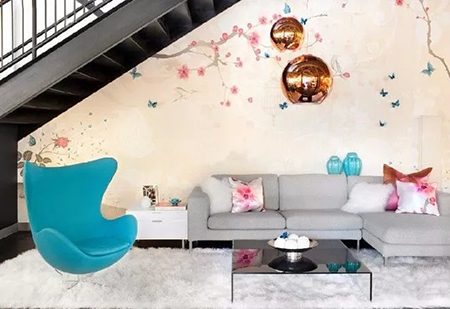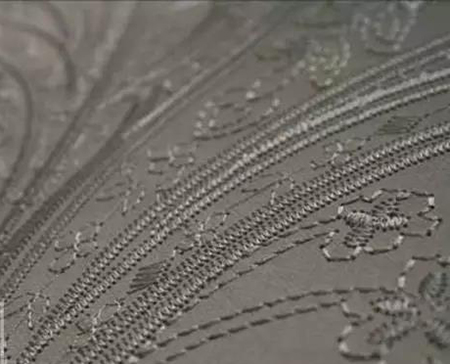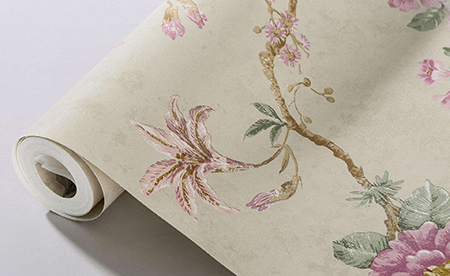|

# 墙纸都有哪些种类?选购难道没有技巧?

之前答应过大家要出关于墙纸和硅藻泥的文章，所以本期就来跟大家聊一下大家比较关心的墙纸。墙纸的优缺点大家都很了解了就不说了，我们今天就聊一下墙纸的分类、价格和选购技巧。墙纸：也称为壁纸、墙布，是一种用于裱糊墙面的室内装修材料。材质不局限于纸，也包含其他材料。因为色彩多样、图案丰富等特点，适用于室内各个空间墙面装饰。

墙纸分类

1、纺织物壁纸/墙布

墙布是以纱布为基底，面层用丝、羊毛、棉、麻等纤维织成织纺及刺绣工艺，PVC压花的比较少。

2.锦缎墙布是更为高级的一种，缎面织有古雅精致的花纹，色泽绚丽多彩，质地柔软，裱糊的技术性和工艺性要求很高。价格贵，属室内高级装饰！

3.pvc（塑料）墙纸

这是市面上最为常见的壁纸同时也是市面上销量最多、销售最快的一种壁纸。是一种非常防水、防潮的材质，清洗也十分的方便，表面若有污渍的出现，只需要使用海绵和毛巾轻轻的擦拭即可，而且这类型的墙纸价格也相对便宜，使用的也非常的广泛，但是环保性差了一些，透气性也很不好。

4．发泡材质壁纸

发泡壁纸用100克／平方米的纸作基材，涂塑300～400克／平方米掺有发泡剂的PVC糊状树脂，印花后再发泡而成。

5.无纺布壁纸

无纺布壁纸延展性好、环保、透气、不易泛黄，但价格也比较高。选择时可以根据自身需求、喜好和房屋特点来挑选。

6.纯纸壁纸纯纸壁纸无异味、环保、透气，但时间久了容易泛黄，不能遇水、不能擦拭。而现在市场上天然加强木浆的壁纸是纯纸系列中品质较高的产品，为优质木浆内加入木纤维丝精制而成的。其强度大于普通纯纸壁纸，也做了一定的防水处理，还可以用湿布擦洗、有防静电、不吸尘等特点。

7.天然材料壁纸

天然效果材质壁纸，它是用草、木材、树叶等制成面层的墙纸，风格古朴自然，素雅大方，生活气息浓厚，给人以返朴归真的感受。如果不是追求特殊风格，一般不会选用这种材料的壁纸。

墙纸价格

大家在某宝某东上看到的大部分都是纸基PVC印花、压花、发泡类壁纸，某宝售价50左右/卷（5.3㎡），有店档次的实体店一般买100~200/卷的都有，判别方法很简单，背面是纸基，然后表面是PVC的发泡或者压花，有点像棉花糖，有些还能看到一些小洞等瑕疵。

另一种流行的是无纺布壁纸，从边缘看过去，能看到有无纺布质感的纤维，摸起来有布的手感，某宝卖100+/卷，实体店国产的100~300/卷，进口的一般200~400+/卷。

还有就是墙布了，现在比较流行定制无缝墙布，价格一般按照平米来算，价格在50~200/每平米，价格主要差在表面的花纹编织的复杂程度。

黏胶有各种带有植物名字的土豆胶、玉米胶、糯米胶，现在还有的墙布申请了一种专利：胶直接就贴在墙布背面，然后用熨斗一样的机器加温贴上去，虽说是专利，百变不离其中。

墙纸选购有哪些技巧？

1、劣质墙纸有异味：真正的环保墙纸是无味的，但如果是采用劣质材料制作，则会有一股刺鼻的气味，如果触近仔细闻就可以闻出来。测试耐磨耐脏性：墙纸的耐磨耐脏性也是不容忽视的一点，建议消费者在选购过程中，可用铅笔在纸上画几画，再用橡皮擦，一般高档墙纸，即使表面有凹凸纹理，也很容易擦干净，反而如果是劣质墙纸，则很容易擦破或擦不干净。

2、测试防水防霉性：因为墙纸是纸质的，很多人都担心它的防水效果，可试倒一滴水在壁纸上，如果等了2～3mm，水还没有渗透，说明这款墙纸的防水不错，反之则说明墙纸的防水性不够。

墙纸使用应该注意什么？

1.有的朋友会说墙纸容易受潮，滋生细菌。修补比较难。如果出现大范围的破损，需要整面换掉，不太容易修补。其实现在墙纸的防水处理技术也比较好了，就算是南方，室内也不会一直潮湿（除了一楼的朋友），潮湿时，开空调干燥调节一下湿度。

2.有的朋友说墙纸用两难就翘脚了，产生翘起的原因有两种，一种是墙纸本身质量有问题，与黏胶结合差而且很容易老化。

第二种也是有点难管控的因素，是师傅的施工过程，首先壁纸要贴的好，需要几个条件。

（１）．边缘的处理细致，胶量均匀溢胶处理及时，最理想的情况就是只有背面有胶，而且厚度尽可能保持一致。

（２）．拼缝大小适中，不会互相交叠，肉眼看也不会明显。

（３）．黏胶干透过程宜慢不宜快，最好关闭门窗闭关３～４天。

3.传统壁纸使用寿命在10年左右，可能有的朋友会觉得觉得不知不耐用。其实根本不用纠结这个，因为用不到10年就会想换了。

以上是小一介绍的墙纸用哪种材料好？墙纸怎么选择？对于墙纸的问题我们已经有了一些了解。当然墙纸在市场上有不少的品牌，我们在购买时，为了注重装修的效果，我们一定要购买质量好的产品，一方面质量有保障，另一方面好的墙纸含的甲醛是比较低的。

`声明：本文由入驻焦点开放平台的作者撰写，除焦点官方账号外，观点仅代表作者本人，不代表焦点立场错误信息举报电话： 400-099-0099，邮箱：jubao@vip.sohu.com，或点此进行意见反馈，或点此进行举报投诉。`A B C D E F G H J K L M N P Q R S T W X Y Z
A - B - C - D - E
• A
• 鞍山
• 安庆
• 安阳
• 安顺
• 安康
• 澳门
• B
• 北京
• 保定
• 包头
• 巴彦淖尔
• 本溪
• 蚌埠
• 亳州
• 滨州
• 北海
• 百色
• 巴中
• 毕节
• 保山
• 宝鸡
• 白银
• 巴州
• C
• 承德
• 沧州
• 长治
• 赤峰
• 朝阳
• 长春
• 常州
• 滁州
• 池州
• 长沙
• 常德
• 郴州
• 潮州
• 崇左
• 重庆
• 成都
• 楚雄
• 昌都
• 慈溪
• 常熟
• D
• 大同
• 大连
• 丹东
• 大庆
• 东营
• 德州
• 东莞
• 德阳
• 达州
• 大理
• 德宏
• 定西
• 儋州
• 东平
• E
• 鄂尔多斯
• 鄂州
• 恩施
F - G - H - I - J
• F
• 抚顺
• 阜新
• 阜阳
• 福州
• 抚州
• 佛山
• 防城港
• G
• 赣州
• 广州
• 桂林
• 贵港
• 广元
• 广安
• 贵阳
• 固原
• H
• 邯郸
• 衡水
• 呼和浩特
• 呼伦贝尔
• 葫芦岛
• 哈尔滨
• 黑河
• 淮安
• 杭州
• 湖州
• 合肥
• 淮南
• 淮北
• 黄山
• 菏泽
• 鹤壁
• 黄石
• 黄冈
• 衡阳
• 怀化
• 惠州
• 河源
• 贺州
• 河池
• 海口
• 红河
• 汉中
• 海东
• 怀来
• I
• J
• 晋中
• 锦州
• 吉林
• 鸡西
• 佳木斯
• 嘉兴
• 金华
• 景德镇
• 九江
• 吉安
• 济南
• 济宁
• 焦作
• 荆门
• 荆州
• 江门
• 揭阳
• 金昌
• 酒泉
• 嘉峪关
K - L - M - N - P
• K
• 开封
• 昆明
• 昆山
• L
• 廊坊
• 临汾
• 辽阳
• 连云港
• 丽水
• 六安
• 龙岩
• 莱芜
• 临沂
• 聊城
• 洛阳
• 漯河
• 娄底
• 柳州
• 来宾
• 泸州
• 乐山
• 六盘水
• 丽江
• 临沧
• 拉萨
• 林芝
• 兰州
• 陇南
• M
• 牡丹江
• 马鞍山
• 茂名
• 梅州
• 绵阳
• 眉山
• N
• 南京
• 南通
• 宁波
• 南平
• 宁德
• 南昌
• 南阳
• 南宁
• 内江
• 南充
• P
• 盘锦
• 莆田
• 平顶山
• 濮阳
• 攀枝花
• 普洱
• 平凉
Q - R - S - T - W
• Q
• 秦皇岛
• 齐齐哈尔
• 衢州
• 泉州
• 青岛
• 清远
• 钦州
• 黔南
• 曲靖
• 庆阳
• R
• 日照
• 日喀则
• S
• 石家庄
• 沈阳
• 双鸭山
• 绥化
• 上海
• 苏州
• 宿迁
• 绍兴
• 宿州
• 三明
• 上饶
• 三门峡
• 商丘
• 十堰
• 随州
• 邵阳
• 韶关
• 深圳
• 汕头
• 汕尾
• 三亚
• 三沙
• 遂宁
• 山南
• 商洛
• 石嘴山
• T
• 天津
• 唐山
• 太原
• 通辽
• 铁岭
• 泰州
• 台州
• 铜陵
• 泰安
• 铜仁
• 铜川
• 天水
• 天门
• W
• 乌海
• 乌兰察布
• 无锡
• 温州
• 芜湖
• 潍坊
• 威海
• 武汉
• 梧州
• 渭南
• 武威
• 吴忠
• 乌鲁木齐
X - Y - Z
• X
• 邢台
• 徐州
• 宣城
• 厦门
• 新乡
• 许昌
• 信阳
• 襄阳
• 孝感
• 咸宁
• 湘潭
• 湘西
• 西双版纳
• 西安
• 咸阳
• 西宁
• 仙桃
• 西昌
• Y
• 运城
• 营口
• 盐城
• 扬州
• 鹰潭
• 宜春
• 烟台
• 宜昌
• 岳阳
• 益阳
• 永州
• 阳江
• 云浮
• 玉林
• 宜宾
• 雅安
• 玉溪
• 延安
• 榆林
• 银川
• Z
• 张家口
• 镇江
• 舟山
• 漳州
• 淄博
• 枣庄
• 郑州
• 周口
• 驻马店
• 株洲
• 张家界
• 珠海
• 湛江
• 肇庆
• 中山
• 自贡
• 资阳
• 遵义
• 昭通
• 张掖
• 中卫

1室1厅1厨1卫1阳台

1
2
3
4
5

0
1
2

1

1

0
1
2
3报名成功，资料已提交审核A B C D E F G H J K L M N P Q R S T W X Y Z
A - B - C - D - E
• A
• 鞍山
• 安庆
• 安阳
• 安顺
• 安康
• 澳门
• B
• 北京
• 保定
• 包头
• 巴彦淖尔
• 本溪
• 蚌埠
• 亳州
• 滨州
• 北海
• 百色
• 巴中
• 毕节
• 保山
• 宝鸡
• 白银
• 巴州
• C
• 承德
• 沧州
• 长治
• 赤峰
• 朝阳
• 长春
• 常州
• 滁州
• 池州
• 长沙
• 常德
• 郴州
• 潮州
• 崇左
• 重庆
• 成都
• 楚雄
• 昌都
• 慈溪
• 常熟
• D
• 大同
• 大连
• 丹东
• 大庆
• 东营
• 德州
• 东莞
• 德阳
• 达州
• 大理
• 德宏
• 定西
• 儋州
• 东平
• E
• 鄂尔多斯
• 鄂州
• 恩施
F - G - H - I - J
• F
• 抚顺
• 阜新
• 阜阳
• 福州
• 抚州
• 佛山
• 防城港
• G
• 赣州
• 广州
• 桂林
• 贵港
• 广元
• 广安
• 贵阳
• 固原
• H
• 邯郸
• 衡水
• 呼和浩特
• 呼伦贝尔
• 葫芦岛
• 哈尔滨
• 黑河
• 淮安
• 杭州
• 湖州
• 合肥
• 淮南
• 淮北
• 黄山
• 菏泽
• 鹤壁
• 黄石
• 黄冈
• 衡阳
• 怀化
• 惠州
• 河源
• 贺州
• 河池
• 海口
• 红河
• 汉中
• 海东
• 怀来
• I
• J
• 晋中
• 锦州
• 吉林
• 鸡西
• 佳木斯
• 嘉兴
• 金华
• 景德镇
• 九江
• 吉安
• 济南
• 济宁
• 焦作
• 荆门
• 荆州
• 江门
• 揭阳
• 金昌
• 酒泉
• 嘉峪关
K - L - M - N - P
• K
• 开封
• 昆明
• 昆山
• L
• 廊坊
• 临汾
• 辽阳
• 连云港
• 丽水
• 六安
• 龙岩
• 莱芜
• 临沂
• 聊城
• 洛阳
• 漯河
• 娄底
• 柳州
• 来宾
• 泸州
• 乐山
• 六盘水
• 丽江
• 临沧
• 拉萨
• 林芝
• 兰州
• 陇南
• M
• 牡丹江
• 马鞍山
• 茂名
• 梅州
• 绵阳
• 眉山
• N
• 南京
• 南通
• 宁波
• 南平
• 宁德
• 南昌
• 南阳
• 南宁
• 内江
• 南充
• P
• 盘锦
• 莆田
• 平顶山
• 濮阳
• 攀枝花
• 普洱
• 平凉
Q - R - S - T - W
• Q
• 秦皇岛
• 齐齐哈尔
• 衢州
• 泉州
• 青岛
• 清远
• 钦州
• 黔南
• 曲靖
• 庆阳
• R
• 日照
• 日喀则
• S
• 石家庄
• 沈阳
• 双鸭山
• 绥化
• 上海
• 苏州
• 宿迁
• 绍兴
• 宿州
• 三明
• 上饶
• 三门峡
• 商丘
• 十堰
• 随州
• 邵阳
• 韶关
• 深圳
• 汕头
• 汕尾
• 三亚
• 三沙
• 遂宁
• 山南
• 商洛
• 石嘴山
• T
• 天津
• 唐山
• 太原
• 通辽
• 铁岭
• 泰州
• 台州
• 铜陵
• 泰安
• 铜仁
• 铜川
• 天水
• 天门
• W
• 乌海
• 乌兰察布
• 无锡
• 温州
• 芜湖
• 潍坊
• 威海
• 武汉
• 梧州
• 渭南
• 武威
• 吴忠
• 乌鲁木齐
X - Y - Z
• X
• 邢台
• 徐州
• 宣城
• 厦门
• 新乡
• 许昌
• 信阳
• 襄阳
• 孝感
• 咸宁
• 湘潭
• 湘西
• 西双版纳
• 西安
• 咸阳
• 西宁
• 仙桃
• 西昌
• Y
• 运城
• 营口
• 盐城
• 扬州
• 鹰潭
• 宜春
• 烟台
• 宜昌
• 岳阳
• 益阳
• 永州
• 阳江
• 云浮
• 玉林
• 宜宾
• 雅安
• 玉溪
• 延安
• 榆林
• 银川
• Z
• 张家口
• 镇江
• 舟山
• 漳州
• 淄博
• 枣庄
• 郑州
• 周口
• 驻马店
• 株洲
• 张家界
• 珠海
• 湛江
• 肇庆
• 中山
• 自贡
• 资阳
• 遵义
• 昭通
• 张掖
• 中卫• 手机• 分享
• 设计
免费设计
• 计算器
装修计算器
• 入驻
合作入驻
• 联系
联系我们
• 置顶
返回顶部